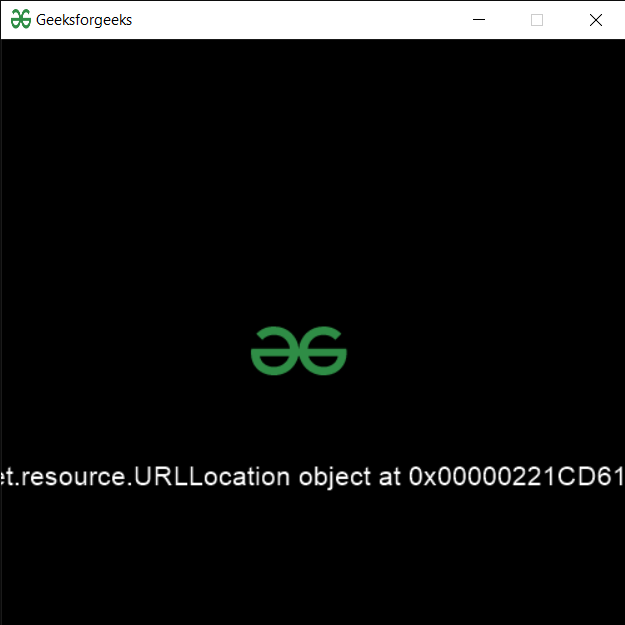Related Articles

# PYGLET – Creating URL Location

• Last Updated : 24 Sep, 2020

In this article we will see how we can create a URL location object in PYGLET module in python. Pyglet is easy to use but powerful library for developing visually rich GUI applications like games, multimedia etc. A window is a “heavyweight” object occupying operating system resources. Windows may appear as floating regions or can be set to fill an entire screen (fullscreen). In order to load a file i.e resource we use resource module of pyglet. This module allows applications to specify a search path for resources. Relative paths are taken to be relative to the application’s __main__ module. URL location object is the location on the network. This class uses the urlparse and urllib2 modules to open files on the network given a URL.

We can create a window object with the help of command given below

```# creating a window
window = pyglet.window.Window(width, height, title)
```

In order to do this we use `URLLocation` method with the pyglet.resource

Syntax : resource.URLLocation(name)

Argument : It takes string i.e base URL as argument

Return : It returns URLLocation object

Below is the implementation

 `# importing pyglet module ``import` `pyglet ``import` `pyglet.window.key as key``   ` `# width of window ``width ``=` `500``   ` `# height of window ``height ``=` `500``   ` `# caption i.e title of the window ``title ``=` `"Geeksforgeeks"``   ` `# creating a window ``window ``=` `pyglet.window.Window(width, height, title) ``   ` `# text  ``text ``=` `"Welcome to GeeksforGeeks"``  ` `# creating label with following proeprties``# font = cooper``# position = 250, 150``# anchor position = center``label ``=` `pyglet.text.Label(text, ``                          ``font_name ``=``'Cooper'``, ``                          ``font_size ``=` `16``, ``                          ``x ``=` `250``,  ``                          ``y ``=` `150``, ``                          ``anchor_x ``=``'center'``,  ``                          ``anchor_y ``=``'center'``)`` ` ` ` `# creating a batch ``batch ``=` `pyglet.graphics.Batch()`` ` `# loading geeksforgeeks image``image ``=` `pyglet.image.load(``'gfg.png'``)`` ` ` ` `# creating sprite object``# it is instance of an image displayed on-screen``sprite ``=` `pyglet.sprite.Sprite(image, x ``=` `200``, y ``=` `230``)``   ` `# on draw event ``@window``.event ``def` `on_draw(): ``       ` `    ``# clear the window ``    ``window.clear() ``       ` `    ``# draw the label``    ``label.draw() ``     ` `    ``# draw the image on screen``    ``sprite.draw()``       ` `# key press event     ``@window``.event ``def` `on_key_press(symbol, modifier): ``   ` `    ``# key "C" get press ``    ``if` `symbol ``=``=` `key.C: ``         ` `        ``# printng the message``        ``print``(``"Key : C is pressed"``)``         ` `# image for icon ``img ``=` `image ``=` `pyglet.resource.image(``"gfg.png"``) `` ` `# setting image as icon ``window.set_icon(img) `` ` ` ` `# creating a url location object``value ``=` `pyglet.resource.URLLocation(``"https://www.geeksforgeeks.org/"``)`` ` `# setting text  of label``label.text ``=` `str``(value)`` ` `# start running the application ``pyglet.app.run() `

Output :Attention geek! Strengthen your foundations with the Python Programming Foundation Course and learn the basics.

To begin with, your interview preparations Enhance your Data Structures concepts with the Python DS Course. And to begin with your Machine Learning Journey, join the Machine Learning – Basic Level Course

My Personal Notes arrow_drop_up